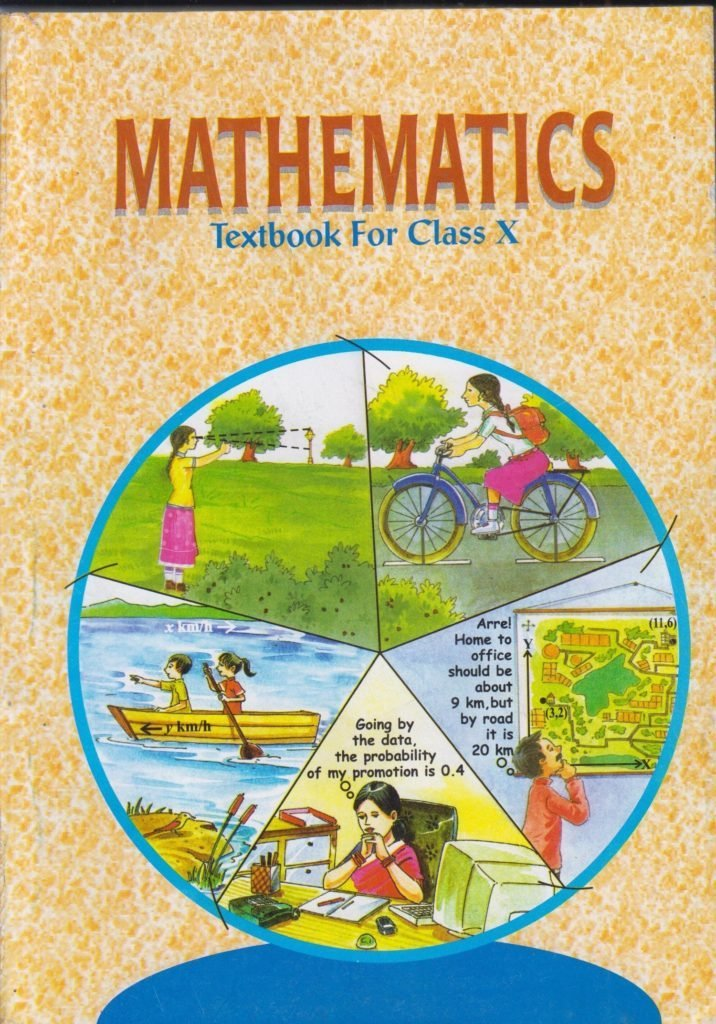Solutions For Class 12 Maths Chapter 1 Relations and Functions, Chapter 2 Inverse Trigonometric Functions, Ch 3 Matrices, Chapter 4 Determinants, Chapter 5 Continuity and Differentiability, Ch 6 Application of Derivatives, Chapter 7 Integrals, Chapter 8 Application of Integrals, Ch 9 Differential Equations, Chapter 10 Vector Algebra, Chapter 11 Three Dimensional Geometry, Ch 12 Linear Programming, Chapter 13 Probability in PDF CBSE, UPSC .